Gauss Law MCQ Level - 2

# Gauss Law MCQ Level - 2 - Class 12

Test Description

## 25 Questions MCQ Test - Gauss Law MCQ Level - 2

Gauss Law MCQ Level - 2 for Class 12 2023 is part of Class 12 preparation. The Gauss Law MCQ Level - 2 questions and answers have been prepared according to the Class 12 exam syllabus.The Gauss Law MCQ Level - 2 MCQs are made for Class 12 2023 Exam. Find important definitions, questions, notes, meanings, examples, exercises, MCQs and online tests for Gauss Law MCQ Level - 2 below.
Solutions of Gauss Law MCQ Level - 2 questions in English are available as part of our course for Class 12 & Gauss Law MCQ Level - 2 solutions in Hindi for Class 12 course. Download more important topics, notes, lectures and mock test series for Class 12 Exam by signing up for free. Attempt Gauss Law MCQ Level - 2 | 25 questions in 50 minutes | Mock test for Class 12 preparation | Free important questions MCQ to study for Class 12 Exam | Download free PDF with solutions
 1 Crore+ students have signed up on EduRev. Have you?
Gauss Law MCQ Level - 2 - Question 1

### In a region with a uniform electric field, the number of lines of force per unit area is E. If a spherical metallic condcutor is placed in the area, the field inside the conductor will be

Detailed Solution for Gauss Law MCQ Level - 2 - Question 1

There is no field inside a metallic conductor  E = 0

Gauss Law MCQ Level - 2 - Question 2

### If one penetrates a uniformly charged spherical cloud electric field strength

Detailed Solution for Gauss Law MCQ Level - 2 - Question 2

In side the sphere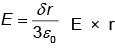therefore field decreases directly as the distance form the centre.

Gauss Law MCQ Level - 2 - Question 3

### An unchanged metal sphere is placed between two equal and oppositely charged metal plates. The nature of lines of force will be

Detailed Solution for Gauss Law MCQ Level - 2 - Question 3

Electric field lines always normal to surface as surface is always equipotential. So option b.

Gauss Law MCQ Level - 2 - Question 4

Consider two concertric spherical surfaces S1 with radius a and S2 with radius 2a, both centred on the origin. There is a charge +q at the origin and no other c harges, Compare the flux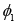through S1 with the flux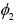through S2 .

Detailed Solution for Gauss Law MCQ Level - 2 - Question 4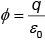as charge is same in both total flux will be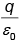for both of them.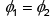Gauss Law MCQ Level - 2 - Question 5

Under what conditions can the electric flux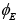be found through a closed surface?

Detailed Solution for Gauss Law MCQ Level - 2 - Question 5

For total flux we need to know the total charge enclosed inside so option 'b' is correct.

Gauss Law MCQ Level - 2 - Question 6

Three charges of q1 = 1 × 10-6 C, q2 = 2 × 10-6 C and q3 = -3 ×10-6 C have been placed as shown, Then the net electric flux will be maximum for the surface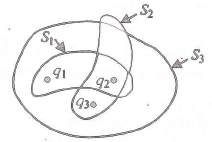Detailed Solution for Gauss Law MCQ Level - 2 - Question 6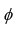will be max if charge enclosed is max S1 has max charge so flux will be max from S1.

Gauss Law MCQ Level - 2 - Question 7

In a region of space, the electric field is given by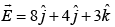. The electric flux through a surface or area of 100 units in x - y plane is

Detailed Solution for Gauss Law MCQ Level - 2 - Question 7

Only field lines normal to surface will cross, so only 2 component will cross 3 ×100 = 300 units.

Gauss Law MCQ Level - 2 - Question 8

A flat square surface with sides of length L is described by the equations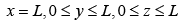The electric flux through the square due to a positive point charge q located at the origin (x = 0, y = 0, z = 0 ) is

Detailed Solution for Gauss Law MCQ Level - 2 - Question 8

As charge is at corner total flux will be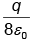, but flux from one face will be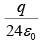.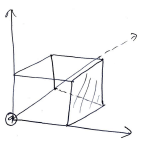Gauss Law MCQ Level - 2 - Question 9

Two conducting plates X and Y, each having large surface area A (on one side) are placed parallel to each other. The plate X is given a charge Q whereas the other is neutral. The electric field at a point in between the plates is given by :

Detailed Solution for Gauss Law MCQ Level - 2 - Question 9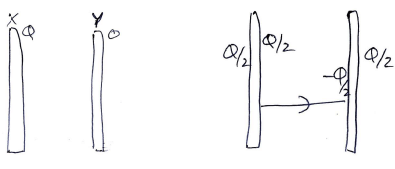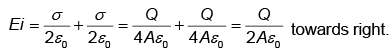Gauss Law MCQ Level - 2 - Question 10

Law stated as flux is 1/Eo times the total charge is

Gauss Law MCQ Level - 2 - Question 11

A hemispherical surface of radius R is placed with its cross-section perpendicular to a uniform elcetric field E as shown in Fig. Flux linked with its curved surface is :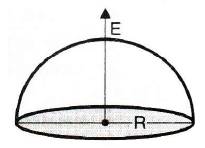Detailed Solution for Gauss Law MCQ Level - 2 - Question 11

As total flux is zero.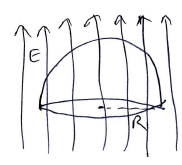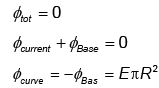Gauss Law MCQ Level - 2 - Question 12

The Fig. shows two parallel equipotential surfaces A and B at same potential kept at a distance r apart from each other. A point cahrge -q is taken from surface A to B, the amount of net work done W will be :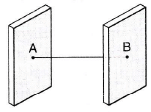Detailed Solution for Gauss Law MCQ Level - 2 - Question 12

As surfaces are equipotential
ΔV = 0
W = qΔV = 0

*Multiple options can be correct
Gauss Law MCQ Level - 2 - Question 13

An ellipsoidal cavity is curved within a perfect conductor. A positive charge q is placed at the centre of the cavity. The points A and B are on the cavity surface as shown in Fig.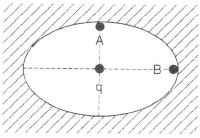Detailed Solution for Gauss Law MCQ Level - 2 - Question 13

Potential will be same at A and B but as field is stranger as sharper edges so B will have stranger field as its radius is smaller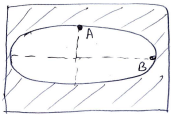Gauss Law MCQ Level - 2 - Question 14

Two thin infinite sheets have uniform surface densities of charge +σ and - σ . Electric field in the space between the two sheets is :

Detailed Solution for Gauss Law MCQ Level - 2 - Question 14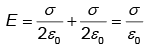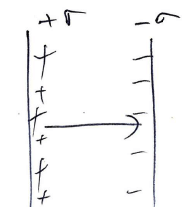Gauss Law MCQ Level - 2 - Question 15

Fig. shows a hemisphere of radius 'R'. A point charge q is kept at distance 'y' above the centre such that y → 0.
Electric flux through the curved surface is :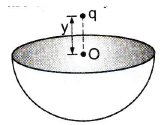Detailed Solution for Gauss Law MCQ Level - 2 - Question 15

As charge may be considered to be at face center, q/2 will be inside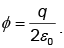Gauss Law MCQ Level - 2 - Question 16

A and B are two concentric spheres, If A is given a charge Q while B is earthed as shown in fig, then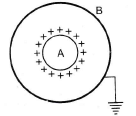Detailed Solution for Gauss Law MCQ Level - 2 - Question 16

Outward charge will flow so field will only be in between A and B outside B or inside A, net charge is zero So E = 0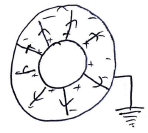Gauss Law MCQ Level - 2 - Question 17

A solid metallic sphere has a charge +3Q concentric with this sphere is a conducting spherical shell having charge -Q. The radius of the sphere is a and that of the spherical shell is b(>a). What is the electric field at a distance r (a <r <b) from the centre?

Detailed Solution for Gauss Law MCQ Level - 2 - Question 17

We don't have to consider charges outside and as point is outside the solid sphere, charges can be considered to be at cneter So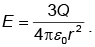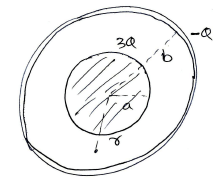Gauss Law MCQ Level - 2 - Question 18

There are two concentric metal shells of radii r1 and r2 (<r1). If the outer shell has a charge q and the inner shell is grounded, the charge on the inner shell is :

Detailed Solution for Gauss Law MCQ Level - 2 - Question 18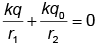as inner shell is grounded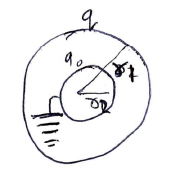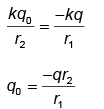Gauss Law MCQ Level - 2 - Question 19

A solid conducting sphere having a charge Q is surrounded by an uncharged concentric conducting hollow spherical shell. Let the potential difference between the surface of the solid sphere and that of the outer surface of the hollow shell be V. If the shell is now given a charge -3Q, the new potential difference between the same two surface is :

Detailed Solution for Gauss Law MCQ Level - 2 - Question 19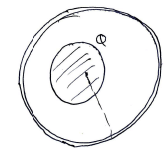If extra charge is given to outer shell there is no charge is P.Δ (discussed in class.)

Gauss Law MCQ Level - 2 - Question 20

A uniformly charged an infinitely long line having a  iinear charge density 'λ' is placed at a normal distance y from a point O, Consider s sphere of radius R with O as centre and R > y. Electric flux through the surface of the sphere is :

Detailed Solution for Gauss Law MCQ Level - 2 - Question 20

Part inside =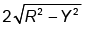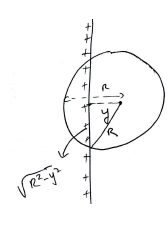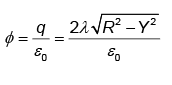Gauss Law MCQ Level - 2 - Question 21

An insulating solid sphere of radius 'R' is charged in a non-uniform manner such that volume charge density P(A/r), where A is a positive constant and r the distance from centre. Electric field strength at any inside point at distance r1 is :

Detailed Solution for Gauss Law MCQ Level - 2 - Question 21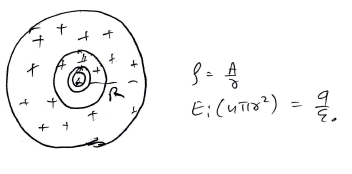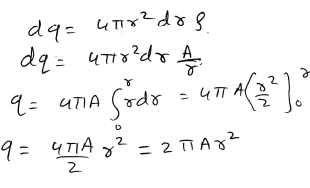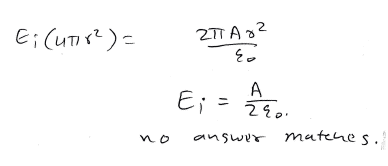Gauss Law MCQ Level - 2 - Question 22

Three infinitely long charge sheets are placed as shown in figure. The electric field at point P is :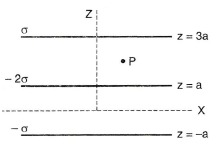Detailed Solution for Gauss Law MCQ Level - 2 - Question 22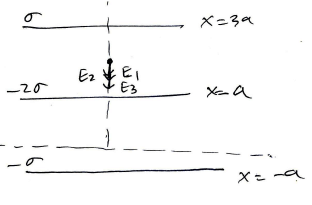All the fields will be directed downwards.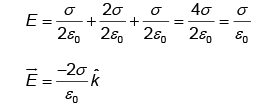Gauss Law MCQ Level - 2 - Question 23

A spherical portion has been removed from a solid sphere having a charge distributed uniformly in its volume as shown in the fig. The electric field inside the emptied space is :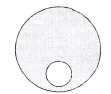Detailed Solution for Gauss Law MCQ Level - 2 - Question 23

E inside is cont (class question)

Gauss Law MCQ Level - 2 - Question 24

Figure shows an imaginary cube of edge L/2. An uniformly charged rod of length L moves towards left at a small but constant speed v. At t = 0, the left end just touches the centre of the face of the cube opposite it. Which of the graphs shown in the figure represents the flux of the electric field through the cube as the rod goes through it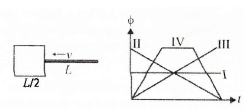Detailed Solution for Gauss Law MCQ Level - 2 - Question 24

Class question.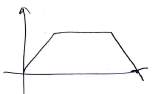Gauss Law MCQ Level - 2 - Question 25

A conducting sphere S1 intersects a closed surfae S2 as shown in the figure. A positive charge q is placed at a point P. What is the value of electric flux through the surface S2?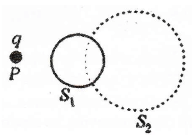Detailed Solution for Gauss Law MCQ Level - 2 - Question 25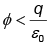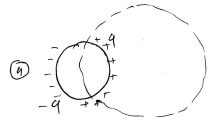As we don't know how much charge will be enclosed.

Information about Gauss Law MCQ Level - 2 Page
In this test you can find the Exam questions for Gauss Law MCQ Level - 2 solved & explained in the simplest way possible. Besides giving Questions and answers for Gauss Law MCQ Level - 2, EduRev gives you an ample number of Online tests for practice(Scan QR code)